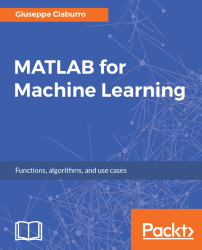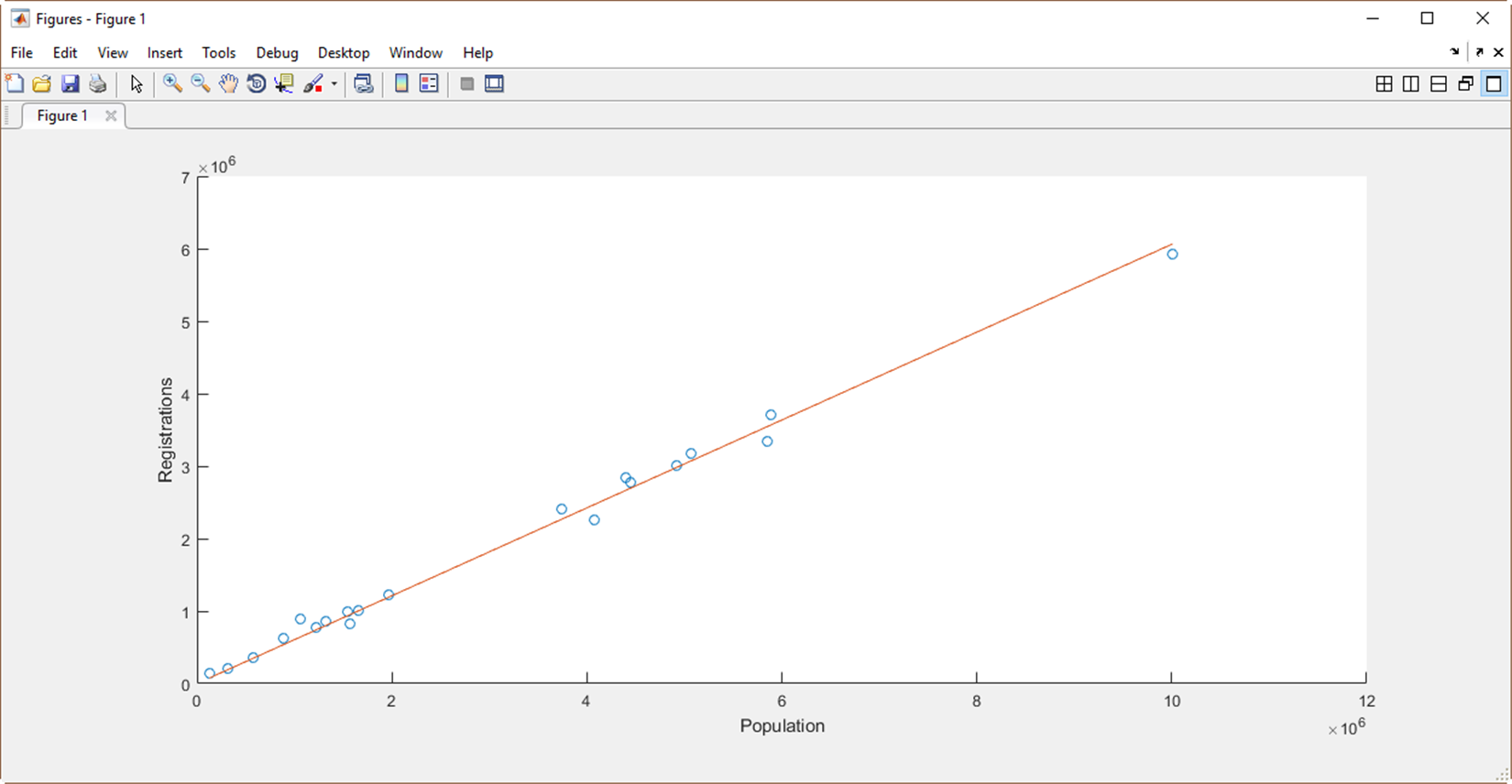•#### MATLAB for Machine Learning#### Overview of this book

MATLAB is the language of choice for many researchers and mathematics experts for machine learning. This book will help you build a foundation in machine learning using MATLAB for beginners. You’ll start by getting your system ready with t he MATLAB environment for machine learning and you’ll see how to easily interact with the Matlab workspace. We’ll then move on to data cleansing, mining and analyzing various data types in machine learning and you’ll see how to display data values on a plot. Next, you’ll get to know about the different types of regression techniques and how to apply them to your data using the MATLAB functions. You’ll understand the basic concepts of neural networks and perform data fitting, pattern recognition, and clustering analysis. Finally, you’ll explore feature selection and extraction techniques for dimensionality reduction for performance improvement. At the end of the book, you will learn to put it all together into real-world cases covering major machine learning algorithms and be comfortable in performing machine learning with MATLAB.
Title PageCreditsForewordwww.PacktPub.comCustomer FeedbackPrefaceFree Chapter
Getting Started with MATLAB Machine LearningImporting and Organizing Data in MATLABFrom Data to Knowledge DiscoveryFinding Relationships between Variables - Regression TechniquesPattern Recognition through Classification AlgorithmsIdentifying Groups of Data Using Clustering MethodsSimulation of Human Thinking - Artificial Neural NetworksImproving the Performance of the Machine Learning Model - Dimensionality ReductionMachine Learning in Practice## Data fitting with neural networks

Data fitting is the process of building a curve or a mathematical function that has the best match with a set of previously collected points. Curve fitting can relate to both interpolations, where exact data points are required, or smoothing, where a flat function that approximates the data is built. We are talking about curves fitting in a regression analysis, which is most concerned with statistical inference problems, as well as the uncertainty that a curve coincides with observed data that has random errors. The approximate curves obtained from the data fitting can be used to help display data, to predict the values of a function where no data is available, and to summarize the relationship between two or more variables.Figure 7.18: Linear interpolation of collected data

We have seen some examples of curve fitting in the regression chapter. On that occasion, we could see that the mathematical formulas that allow us to predict the trend of a particular...